# Reason - math word problems

1. Bookshelf and books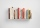How many ways can we place 7 books in a bookshelf?
2. Dog price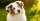Tereza agreed to get a dog and 6000 crowns a year for help in a dachshund breeding station. After eight months she had to finish work and got a dog and 2000 crowns. What price does a dog have?
3. Probability of intersectionThree students have a probability of 0.7,0.5 and 0.4 to graduated from university respectively. What is the probability that at least one of them will be graduated?
4. Friction coefficientWhat is the weight of a car when it moves on a horizontal road at a speed of v = 50 km/h at engine power P = 7 kW? The friction coefficient is 0.07
5. Iron pole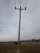The iron pole is in the ground 2/5 of its length, partly above the ground 1/3 is yellow, and the unpainted section is 6 m long. How long is the entire column?
6. Barter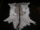There is exchange trade on the market. We know that for two sheepskins we get three goat skins. We also know that for six rabbits skins we get four goat skins. How many rabbit's skins we get for four sheepskins?
7. Dance groupThe dance group formed groups of 4, 5, and 6 members. Always one dancer remains. How many dancers were there in the whole group?
8. A jackpot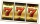How many times must I play this jackpot to win? A jackpot of seven games having (1 X 2), i. E. , home win or away win.
9. Uphill and downhillThe cyclist moves uphill at a constant speed of v1 = 10 km/h. When he reaches the top of the hill, he turns and passes the same track downhill at a speed of v2 = 40 km/h. What is the average speed of a cyclist?
10. Last pageTwo consecutive sheets dropped out of the book. The sum of the numbers on the sides of the dropped sheets is 154. What is the number of the last page of the dropped sheets?
11. Four paversFour pavers would pave the square in 18 days. How many pavers do you need to add to done work in 12 days?
12. Centroid - two bodiesA body is composed of a 0.8 m long bar and a sphere with a radius of 0.1m attached so that its center lies on the longitudinal axis of the bar. Both bodies are of the same uniform material. The sphere is twice as heavy as the bar. Find the center of gravi
13. The workThe work was to be done by 150 workers. At the beginning of their work, their number reduced by 40, which increased the time of work by 5 and 1/3 of the schedule. How long did work take?
14. The King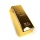The King wants to divide his sons equally. He has 42 rubies and 45 diamonds. How many sons and how will they share them?
15. Luggage and air travelTwo friends traveling by plane had a total of 35 kg of luggage. They paid one 72 CZK and second 108 CZK for being overweight. If only one paid for all the bags, it would cost 300 CZK. What weight of baggage did each of them have, how many kilograms of lug
16. Cows and calves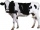There are 168 cows and calves in the cowshed. Cows are in nine stalls and calves in four stalls. Same count cows are in every cow stall and three more in each calf stall than in a cow stall. What is the capacity of the stalls for cows and what for calves?
17. Candies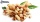In the confectionery, the price for 1 kg of pistachio candies cost CZK 360, and the price for 1 kg of hazelnut candies was CZK 280. Mixing these two types of sweets created a box of chocolates. How many grams of pistachios and how many grams of hazelnut c
18. Ten boys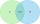Ten boys chose to go to the supermarket. Six boys bought gum and nine boys bought a lollipop. How many boys bought gum and a lollipop?
19. 600 pencils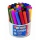600 pencils we want to be divided into three groups. The biggest groups have ten pens more than the smallest. How many ways can this be done?
20. Boys and girlsThere are 11 boys and 18 girls in the classroom. Three pupils will answer. What is the probability that two boys will be among them?

Do you have an interesting mathematical word problem that you can't solve it? Submit math problem, and we can try to solve it.

We will send a solution to your e-mail address. Solved examples are also published here. Please enter the e-mail correctly and check whether you don't have a full mailbox.

Please do not submit problems from current active competitions such as Mathematical Olympiad, correspondence seminars etc...